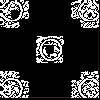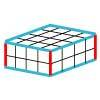#### You may also like### Doplication

We can arrange dots in a similar way to the 5 on a dice and they usually sit quite well into a rectangular shape. How many altogether in this 3 by 5? What happens for other sizes?### Round and Round the Circle

What happens if you join every second point on this circle? How about every third point? Try with different steps and see if you can predict what will happen.### Making Cuboids

Let's say you can only use two different lengths - 2 units and 4 units. Using just these 2 lengths as the edges how many different cuboids can you make?

# Counting Cogs

##### Age 7 to 14Challenge Level

This was a tricky problem but we received some detailed solutions - well done.

Frank, Noah and Ben from Stanford Junior School said:

If the number of teeth on one cog is the multiple of teeth on the other cog it will not work. So if the teeth on one cog is 5 and on the other cog is ten teeth it will not work.
Also the number you times the teeth to make the number of teeth on the other cog that is the number of spaces it goes into.
cog $1$: $5$ teeth
cog $2$: $10$ teeth
spaces gone into = $2$
$5 \times 2 =10$

Sean, Ben, Lane and Jonathan from Meridian Primary School told us about some of the things they noticed:

First of all, all consecutive numbers will work because the smaller will go round once but won't make it to the start point by one so eventually it will make it the whole way round (e.g. $4$, $5$).
Two even numbers will never ever go together because they are multiples of two so they will keep going in a rut.
If two odd numbers are factors of two consecutive numbers they will work because they'll still act as consecutive numbers would e.g. $5$ and $7$:
$5 \times 3 = 15$ and $7 \times 2 = 14$
$11$ works with all numbers.

Pranjal from Garden International School, Kuala Lumpur took these ideas a bit further and wrote:

There are three different ways of determining which pairs of cogs work and which don't:

1. Find consecutive numbers from $4$ to $12$ and pair them up. The solutions that are in this category are as follows:
$4$-$5$, $5$-$6$, $6$-$7$, $7$-$8$, $8$-$9$, $9$-$10$, $10$-$11$ and $11$-$12$

2. Make pairs of odd numbers. The pairs are:
$5$-$7$, $5$-$9$, $5$-$11$, $7$-$9$, $7$-$11$ and $9$-$11$

3. The last, and the category that most numbers fall into, is the HCF (highest common factor) category. This category also includes the pairs in the previous category. The rule is that the numbers have to have a HCF of $1$. All the numbers that work are in this category too.

The pairs that go together are:
$4$-$5$, $5$-$6$, $6$-$7$, $7$-$8$, $8$-$9$, $9$-$10$, $10$-$11$, $11$-$12$, $5$-$7$, $5$-$9$, $5$-$11$, $7$-$9$, $7$-$11$, $6$-$11$, $7$-$10$, $5$-$8$, $9$-$11$, $4$-$7$, $4$-$9$, $4$-$11$, $5$-$12$, $6$-$11$, $8$-$11$

Note: Even-Even numbers can never go together, no matter what the case. This is because their one factor is definitely $2$, if not the highest. Some particular odd-even numbers can't go together either. Examples are $5$-$10$ and $9$-$12$.The rules are that there cannot be a pair which consists of numbers which are both divisible by a certain number other than one or that one number is divisible by the other number in the pair.

QUESTION
We had to ask ourselves a question as we progressed in the solution. The question was that which pairs work and why, and is there a pattern between the pairs that work.

Jong Woong, Jayme, Denise and Mariana, also from Garden International School, have reasoned through this problem very carefully. They told us how they worked to solve the problem. First, they said they wrote down all the pairs possible using the $4$, $5$, $6$, $7$, $8$, $10$, $11$ and $12$ cogs:

($4$, $5$) ($4$, $6$) ($4$, $7$) ($4$, $8$) ($4$, $10$) ($4$, $11$) ($4$, $12$)
($5$, $6$) ($5$, $7$) ($5$, $8$) ($5$, $10$) ($5$, $11$) ($5$, $12$)
($6$, $7$) ($6$, $8$) ($6$, $10$) ($6$, $11$) ($6$, $12$)
($7$, $8$) ($7$, $10$) ($7$, $11$) ($7$, $12$)
($8$, $10$) ($8$, $11$) ($8$, $12$)
($10$, $11$) ($10$, $12$) ($11$, $12$)

Then they tried random cogs to see if they worked and tried to find a pattern among them.

Pairs that work
($4$, $5$) ($4$, $7$) ($4$, $11$) ($5$, $6$) ($5$, $7$) ($5$, $11$) ($5$, $12$) ($6$, $7$) ($6$, $11$) ($7$, $8$) ($7$, $10$) ($7$, $11$) ($7$, $12$) ($8$, $11$) ($10$, $11$) ($11$, $12$)

Pairs that don't work
($4$, $6$) ($4$, $8$) ($4$, $10$) ($4$, $12$) ($5$, $8$) ($5$, $10$) ($6$, $8$) ($6$, $10$) ($6$, $12$) ($8$, $10$) ($8$, $12$) ($10$, $12$)

We found out that that the pairs that have a HCF (highest common factor) of $1$ work.
This only happens if the highest common factor is one, because the cogs get filled in by the highest common factor. For example, the pair ($4$, $6$) doesn't work because the HCM is $2$ which means that the space will be filled in by '$2$'.
Well done to all of you who sent a solution to this problem. I wonder whether anyone else can find a way to explain why cogs will only work if they have a HCF of $1$?